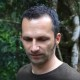author Manuel M T ChakravartyMon, 7 Jun 2010 11:05:33 +0000 (11:05 +0000) committer Manuel M T ChakravartyMon, 7 Jun 2010 11:05:33 +0000 (11:05 +0000)

index 627586b..a15f32d 100644 (file)
@@ -1,4 +1,4 @@
--- This program is heavily GC bound unless we use a very large heap (eg, -H500M)
+-- For lists, this program is heavily GC bound unless we use a very large heap (eg, -H500M)

import GHC.Conc (par, pseq)

@@ -9,12 +9,60 @@ import System.CPUTime
import System.Time
import System.Random

+import Data.Sequence (Seq, (<|), (><))
+import qualified Data.Sequence as Seq
+import qualified Data.Foldable as Fold
+
import qualified Data.Array.Parallel.Unlifted as U

-- Time
--

+data Time = Time { cpu_time  :: Integer
+                 , wall_time :: Integer
+                 }
+
+type TimeUnit = Integer -> Integer
+
+picoseconds :: TimeUnit
+picoseconds = id
+
+milliseconds :: TimeUnit
+milliseconds n = n `div` 1000000000
+
+seconds :: TimeUnit
+seconds n = n `div` 1000000000000
+
+cpuTime :: TimeUnit -> Time -> Integer
+cpuTime f = f . cpu_time
+
+wallTime :: TimeUnit -> Time -> Integer
+wallTime f = f . wall_time
+
+getTime :: IO Time
+getTime =
+  do
+    cpu          <- getCPUTime
+    TOD sec pico <- getClockTime
+    return \$ Time cpu (pico + sec * 1000000000000)
+
+zipT :: (Integer -> Integer -> Integer) -> Time -> Time -> Time
+zipT f (Time cpu1 wall1) (Time cpu2 wall2) =
+  Time (f cpu1 cpu2) (f wall1 wall2)
+
+minus :: Time -> Time -> Time
+minus = zipT (-)
+
+fromTime :: Time -> (Integer, Integer)
+fromTime t = (wallTime milliseconds t, cpuTime milliseconds t)
+
+instance Show Time where
+  showsPrec n t = showsPrec n (wallTime milliseconds t)
+                . showChar '/'
+                . showsPrec n (cpuTime milliseconds t)
+

-- Random points generation
--
@@ -61,43 +109,46 @@ instance Show Point where
nf (Point x y:xs) = x `seq` y `seq` nf xs
nf []             = ()

+distance :: Point -> Line -> Double
+distance (Point xo yo) (Line (Point x1 y1) (Point x2 y2))
+  = (x1-xo) * (y2 - yo) - (y1 - yo) * (x2 - xo)
+
+
+-- Sequential list version
+
upper :: (a -> a -> Bool) -> [(a, b)] -> b
upper above = snd . foldl1 pick
where
pick left@(kl, _) right@(kr, _) | kl `above` kr = left
| otherwise     = right

-distance :: Point -> Line -> Double
-distance (Point xo yo) (Line (Point x1 y1) (Point x2 y2))
-  = (x1-xo) * (y2 - yo) - (y1 - yo) * (x2 - xo)
-
-hsplit :: [Point] -> Line -> [Point]
-hsplit points line@(Line p1 p2)
+hsplitList :: [Point] -> Line -> [Point]
+hsplitList points line@(Line p1 p2)
| length packed < 2 = p1:packed
-  | otherwise         = hsplit packed (Line p1 pm) ++ hsplit packed (Line pm p2)
+  | otherwise         = hsplitList packed (Line p1 pm) ++ hsplitList packed (Line pm p2)
where
cross  = [ (distance p line, p) | p <- points ]
packed = [ p | (p, (c, _)) <- zip points cross, c > 0.0 ]

pm     = upper (>) cross

-quickHull :: [Point] -> [Point]
-quickHull [] = []
-quickHull points
-  = hsplit points (Line minx maxx) ++ hsplit points (Line maxx minx)
+quickHullList :: [Point] -> [Point]
+quickHullList [] = []
+quickHullList points
+  = hsplitList points (Line minx maxx) ++ hsplitList points (Line maxx minx)
where
xs   = [ (x, p) | p@(Point x y) <- points ]
minx = upper (<) xs
maxx = upper (>) xs

--- Parallel version
+-- Parallel list version

-hsplitPar :: [Point] -> Line -> [Point]
-hsplitPar points line@(Line p1 p2)
+hsplitListPar :: [Point] -> Line -> [Point]
+hsplitListPar points line@(Line p1 p2)
| length packed < 2 = p1:packed
-  | otherwise         = let left  = hsplitPar packed (Line p1 pm)
-                            right = hsplitPar packed (Line pm p2)
+  | otherwise         = let left  = hsplitListPar packed (Line p1 pm)
+                            right = hsplitListPar packed (Line pm p2)
in
right `par`
(left ++ right)
@@ -107,11 +158,11 @@ hsplitPar points line@(Line p1 p2)

pm     = upper (>) cross

-quickHullPar :: [Point] -> [Point]
-quickHullPar [] = []
-quickHullPar points
-  = let left  = hsplitPar points (Line minx maxx)
-        right = hsplitPar points (Line maxx minx)
+quickHullListPar :: [Point] -> [Point]
+quickHullListPar [] = []
+quickHullListPar points
+  = let left  = hsplitListPar points (Line minx maxx)
+        right = hsplitListPar points (Line maxx minx)
in
right `par`
(left ++ right)
@@ -125,6 +176,65 @@ quickHullPar points
--   slower.  (Keep in mind that even in the good case, this program spends 2/3 of its running time
--   in the GC.)

+
+-- Sequential finger-tree version
+
+upperSeq :: (a -> a -> Bool) -> Seq (a, b) -> b
+upperSeq above = snd . Fold.foldl1 pick
+  where
+    pick left@(kl, _) right@(kr, _) | kl `above` kr = left
+                                    | otherwise     = right
+
+hsplitSeq :: Seq Point -> Line -> Seq Point
+hsplitSeq points line@(Line p1 p2)
+  | Seq.length packed < 2 = p1<|packed
+  | otherwise             = hsplitSeq packed (Line p1 pm) >< hsplitSeq packed (Line pm p2)
+  where
+    cross  = fmap (\p -> (distance p line, p)) points
+    packed = fmap fst \$ Seq.filter (\(p, (c, _)) -> c > 0.0) (Seq.zip points cross)
+
+    pm     = upperSeq (>) cross
+
+quickHullSeq :: Seq Point -> Seq Point
+quickHullSeq points
+  | Seq.null points = points
+  | otherwise       = hsplitSeq points (Line minx maxx) >< hsplitSeq points (Line maxx minx)
+  where
+    xs   = fmap (\p@(Point x y) -> (x, p)) points
+    minx = upperSeq (<) xs
+    maxx = upperSeq (>) xs
+
+
+-- Parallel finger-tree version
+
+hsplitSeqPar :: Seq Point -> Line -> Seq Point
+hsplitSeqPar points line@(Line p1 p2)
+  | Seq.length packed < 2 = p1<|packed
+  | otherwise             = let left  = hsplitSeqPar packed (Line p1 pm)
+                                right = hsplitSeqPar packed (Line pm p2)
+                            in
+                            right `par`
+                            (left >< right)
+  where
+    cross  = fmap (\p -> (distance p line, p)) points
+    packed = fmap fst \$ Seq.filter (\(p, (c, _)) -> c > 0.0) (Seq.zip points cross)
+
+    pm     = upperSeq (>) cross
+
+quickHullSeqPar :: Seq Point -> Seq Point
+quickHullSeqPar points
+  | Seq.null points = points
+  | otherwise       = let left  = hsplitSeqPar points (Line minx maxx)
+                          right = hsplitSeqPar points (Line maxx minx)
+                      in
+                      right `par`
+                      (left >< right)
+  where
+    xs   = fmap (\p@(Point x y) -> (x, p)) points
+    minx = upperSeq (<) xs
+    maxx = upperSeq (>) xs
+
+
-- main
--

@@ -142,9 +252,12 @@ main
oneRun pts = do
t1 <- getTime
let res = case mode of
-                                     "seq" -> quickHull pts
-                                     "par" -> quickHullPar pts
-                                     _     -> error "mode must be 'seq' or 'par'"
+                                     "seq-list" -> quickHullList pts
+                                     "par-list" -> quickHullListPar pts
+                                     "seq-seq"  -> Fold.toList . quickHullSeq    . Seq.fromList \$ pts
+                                     "par-seq"  -> Fold.toList . quickHullSeqPar . Seq.fromList \$ pts
+                                     _          -> error "mode must be 'seq-list', 'par-list', \
+                                                         \'seq-seq', or 'par-seq'"
evaluate \$ nf res
t2 <- getTime
return (length res, fromTime (t2 `minus` t1))